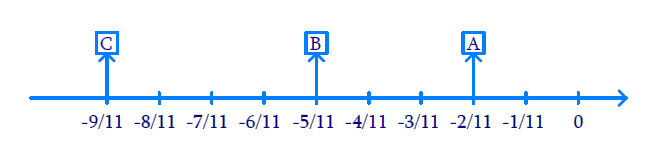# Ex.1.2 Q2 Rational Numbers Solution - NCERT Maths Class 8

## Question

Represent \begin{align}\frac{{ - 2}}{{11}},\;\;\frac{{ - 5}}{{11}},\;\;\frac{{ - 9}}{{11}}\end{align}  on the number line.

Video Solution
Rational Numbers
Ex 1.2 | Question 2

## Text Solution

Reasoning:

The negative numbers are represented on the left of $$0$$ in the number line. The denominator of the rational number indicates the number of equal parts into which the first unit has to be divided whereas the numerator indicates as to how many of these parts are to be taken into consideration.

Steps:

We make $$9$$ marking of distance \begin{align}\frac{1}{{11}}\end{align} each on the left of $$0$$ and starting from $$0.$$

The second marking represent \begin{align}\frac{{ - 2}}{{11}}\end{align} which is represented as $$A$$ in the line.

The fifth marking represent \begin{align}\frac{{ - 5}}{{11}}\end{align} representations $$B$$ in the line.

The ninth marking represent \begin{align}\frac{{ - 9}}{{11}}\end{align} represented as $$C$$ in the number line.Learn from the best math teachers and top your exams

• Live one on one classroom and doubt clearing
• Practice worksheets in and after class for conceptual clarity
• Personalized curriculum to keep up with school